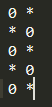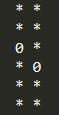17南宁区域赛 J - Rearrangement 【规律】

https://nanti.jisuanke.com/t/19976

0 要摆放的话 肯定是错位摆放的 比如这样AC代码

#pragma comment(linker, "/STACK:102400000,102400000")

#include <cstdio>
#include <cstring>
#include <ctype.h>
#include <cstdlib>
#include <cmath>
#include <climits>
#include <ctime>
#include <iostream>
#include <algorithm>
#include <deque>
#include <vector>
#include <queue>
#include <string>
#include <map>
#include <stack>
#include <set>
#include <list>
#include <numeric>
#include <sstream>
#include <iomanip>
#include <limits>

#define pb push_back
#define fi first
#define se second
#define L(on) ((on)<<1)
#define R(on) (L(on) | 1)
#define mkp(a, b) make_pair(a, b)
#define bug puts("***bug***");
#define all(x) x.begin(), x.end()
#define rall(x) x.rbegin(), x.rend()
#define CLR(a, b) memset(a, (b), sizeof(a));
#define syn_close ios::sync_with_stdio(false); cin.tie(0);
#define sp system("pause");
//#define gets gets_s

using namespace std;

typedef long long ll;
typedef long double ld;
typedef long double ld;
typedef unsigned long long ull;
typedef pair <int, int> pii;
typedef pair <ll, ll> pll;
typedef vector <int> vi;
typedef vector <ll> vll;
typedef vector < vi > vvi;

const double PI = acos(-1.0);
const double EI = exp(1.0);
const double eps = 1e-8;

{
char c = getchar(); int ans = 0, vis = 1;
while (c < '0' || c > '9') { if (c == '-') vis = -vis;  c = getchar(); }
while (c >= '0' && c <= '9') { ans = ans * 10 + c - '0'; c = getchar(); }
return ans * vis;
}

const int INF = 0x3f3f3f3f;
const ll INFLL = 0x3f3f3f3f3f3f3f3fll;
const int maxn = (int)1e2 + 10;
const int MAXN = (int)1e4 + 10;
const ll MOD = (ll)1e9 + 7;

int n;
int arr;

void input()
{
CLR(arr, 0);
for (int i = 0; i < 2 * n; i++)
}

bool solve()
{
if (arr > n) return false;
if (arr <= 1 && arr && arr) return false;
if (arr == 2 && (arr % 2 == 0) && (arr % 2 == 0)) return false;
return true;
}

int main()
{
}